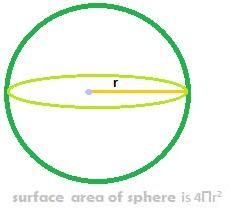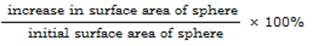## Surface area of a sphere.The total surface area of a sphere or simply the surfacea area of a sphere is 4Πr2

Solved Problems on surface area of a sphere

Example:

The surface area of a sphere is 2464 sq.cm s. Find the radius of the sphere.

Solution:

Surface area of a sphere is 4Πr2

So, 4Πr2 = 2464, i.e.

4 × 22/7 × r2 = 2464, so,

r2 = (2464 × 7)/88 = 196

so, r = √196 = 14

so radius of the sphere is 14 cm's.

Example:

Find the percentage increase in the surface area of a sphere, if the radius of the sphere increases by 50%

Solution:

The surface area of a sphere is 4Πr2

After 50% increase in radius of the sphere, the new radius will become
(150/100)r  = 1.5r or 3r/2,

Substituting this in 4Πr2, we get

4Π×(3r/2)2 = 4Π (9r2/4) = (9/4)( 4Πr2)

So, the final surface area of a sphere, after its radius increases by 50%, will become
(9/4)( 4Πr2)

Now, the amount of increase in the surface area of sphere is:

(9/4)( 4Πr2) – (4Πr2) = (5/4)(4Πr2) = 1.25 × 4Πr2

Therefore, percentage increase in surface area of sphere is(1.25 × 4Πr2) × 100%/( 4Πr2) =

1.25 × 100% = 125%

So, the surface area of a sphere increases by 125%, if the radius of the sphere increases by 50%